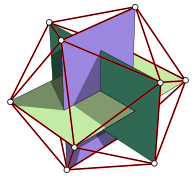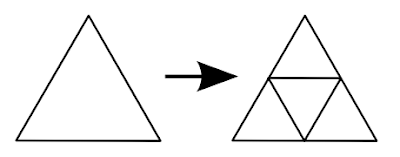*Note: In every code and snippet I use the following self-made math library for vectors and matrices. If you want to use it, then set the following link as resource in your pen. If you use it, then please credit me

If you're a 3d modeller, then you probably used spheres in your scenes. There are 2 main ways you can generate a sphere.

## Cartographers' dream - The UV sphere

Don't let the name of this object throw you off, it's a hell on earth when you try to create a UV map for a sphere. This is true for both cases.

The UV sphere is useful, if you want simmetry or you want to make a cartographical globe. The sphere's surface is divided into rectangles with latitude and longitude lines.

To define a UV sphere you need to know the radius of the sphere and the amount of latitude and longitude segments. To create the vertices, you simply create a plane and wrap it around a sphere.

Additional information: We will be using 3d trigonometry for the sphere. To convert angles around a center point into 3d coordinates, you need to use the following formula:

```  ```x = r * sin(angleY) * cos(angleX)
y = r * sin(angleY) * sin(angleX)
z = r * cos(angleY)
```
```
```  ```var vertices = [];

var latitudeCount = 30;
var longitudeCount = 30;
for (var y = 0; y < longitudeCount; y++) {
for (var x = 0; x < latitudeCount; x++) {
var angleX = 2 * Math.PI / latitudeCount * x;
var angleY = 2 * Math.PI / longitudeCount * y;
var xx = radius * Math.sin(angleY) * Math.cos(angleX);
var yy = radius * Math.sin(angleY) * Math.sin(angleX);
var zz = radius * Math.cos(angleY);
vertices.push(new Vector3(xx, yy, zz));
}
}
```
```

To create the indices, you need to do the same thing as for the tube, except that you need to connect the last vertices vertically too.

```  ```// Create the quads between the vertices
for (var x = 0; x < longitudeCount; x++) {
for (var y = 0; y < latitudeCount; y++) {
var left = x;
var right = (x + 1) % longitudeCount;
var top = y;
var bottom = (y + 1) % latitudeCount;
indices.push(
left + top * longitudeCount,       // vertex at (x; y)
left + bottom * longitudeCount,    // vertex at (x; y + 1)
right + top * longitudeCount,      // vertex at (x + 1; y)

right + top * longitudeCount,      // vertex at (x + 1; y)
left + bottom * longitudeCount,    // vertex at (x; y + 1)
right + bottom * longitudeCount    // vertex at (x + 1; y + 1)
);
}
}
```
```

If you render it you get the following sphere:

## Be a bit less rectangular - The icosphere

The icosphere is a sphere wich consist of triangles. It's generated by subdividing an icosahedron. It's widely used in modelling as it provides a more natural shape and doesn't require preprocessing to use in rendering (like triangulation).

The vertices of an icosahedron are the corners of 3 ortoghonal rectangles:If the rectangle's length is 1, then the height of it is the golden ratio (`(1 + sqrt(5)) / 2` = 1.618...).

In code this look like:

```  ```var vertices = [];
var r = 100;
var ration = (1 + Math.sqrt(5)) / 2 * r;

vertices.push(new Vector3(-r, ration, 0));
vertices.push(new Vector3(r, ration, 0));
vertices.push(new Vector3(-r, -ration, 0));
vertices.push(new Vector3(r, -ration, 0));

vertices.push(new Vector3(0, -r, ration));
vertices.push(new Vector3(0, r, ration));
vertices.push(new Vector3(0, -r, -ration));
vertices.push(new Vector3(0, r, -ration));

vertices.push(new Vector3(ration, 0, -r));
vertices.push(new Vector3(ration, 0, r));
vertices.push(new Vector3(-ration, 0, -r));
vertices.push(new Vector3(-ration, 0, r));
```
```

Creating the indices is again a very hard-code-y process. The indices for an icosahedron are:

```  ```var indices = [
0, 11, 5, 0, 5, 1, 0, 1, 7, 0, 7, 10, 0, 10, 11,
1, 5, 9, 5, 11, 4, 11, 10, 2, 10, 7, 6, 7, 1, 8,
3, 9, 4, 3, 4, 23, 2, 6, 3, 6, 8, 3, 8, 9,
4, 9, 5, 2, 4, 11, 6, 2, 10, 8, 6, 7, 9, 8, 1
];
```
```

### Subdividing the icosahedron

If you render the vertices above, then you get an icosahedron, but it's still not an icosphere. To get an icosphere you need to subdivide it. To subdivide th triangles of the icosahedron you need to divide them into 4 equal triangles, normalize the new vertices and multiply it with the radius.In code

```  ```function subdivide() {
var newVertices = [];
var newIndices = [];
for (var i = 0; i < indices; i += 3) {
var v1 = vertices[indices[i]];
var v2 = vertices[indices[i + 1]];
var v3 = vertices[indices[i + 2]]

v12.normalize().multiply(r);
v23.normalize().multiply(r);
v31.normalize().multiply(r);

var ind = newVertices.length;
newVertices.push(v1, v2, v3, v12, v23, v31);
newIndices.push(ind, ind + 3, ind + 5);     // v1, v12, v31
newIndices.push(ind + 1, ind + 3, ind + 4); // v2, v12, v23
newIndices.push(ind + 2, ind + 4, ind + 5); // v3, v23, v31
newIndices.push(ind + 3, ind + 4, ind + 5); // v12, v23, v31
}
vertices = newVertices;
indices = newIndices;
}
```
```

If you use the above code with the icosahedron generation and you render it, then you get the following:

Note: I made this pen before I created this post series. It contains some additional stuff, like lighting.